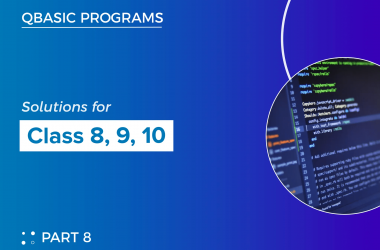# Arithmetic Operators in QBASIC

## Introduction:

QBASIC is a versatile programming language known for its strong set of tools for various computer tasks. One of its main strengths is its arithmetic operators, which allow developers to perform many different types of math operations easily. This blog explores these arithmetic operators in QBASIC and shows how they can be used with clear code examples.

## Arithmetic Operators in QBASIC:

Arithmetic operators are like special symbols that let you do basic math with numbers. QBASIC is great at handling these operators, including addition, subtraction, multiplication, and division. This makes QBASIC a perfect place to play around with math. In this blog, we’ll look at each operator and show you examples of how they work with code to help you understand.

The addition operator (+) gracefully combines two numeric values, returning their sum.
``````   ' Example: Adding two numbers
CLS
DIM a, b, result AS INTEGER
a = 10
b = 20
result = a + b
PRINT "Sum:", result``````
1. Subtraction (-):
Employ the subtraction operator (-) to calculate the difference between two numbers.
``````   ' Example: Subtracting two numbers
CLS
DIM x, y, difference AS INTEGER
x = 50
y = 30
difference = x - y
PRINT "Difference:", difference``````
1. Multiplication ():
The multiplication operator (
) deftly computes the product of two numbers.
``````   ' Example: Multiplying two numbers
CLS
DIM num1, num2, product AS INTEGER
num1 = 8
num2 = 5
product = num1 * num2
PRINT "Product:", product``````
1. Division (/):
For division, the operator (/) divides one number by another, yielding the quotient.
``````   ' Example: Dividing two numbers
CLS
DIM dividend, divisor, quotient AS SINGLE
dividend = 36
divisor = 6
quotient = dividend / divisor
PRINT "Quotient:", quotient``````
1. Modulus (%):
The modulus operator (%) calculates the remainder of a division operation.
``````   ' Example: Modulus operator
CLS
DIM numA, numB, remainder AS INTEGER
numA = 23
numB = 7
remainder = numA MOD numB
PRINT "Remainder:", remainder``````
1. Exponentiation (^):
Employ the exponentiation operator (^) to raise a number to a specified power.
``````   ' Example: Exponentiation operator
CLS
DIM base, exponent, result AS DOUBLE
base = 2
exponent = 3
result = base ^ exponent
PRINT "Result:", result``````

## Conclusion:

Arithmetic operators are like the foundation of math in QBASIC programs. When you know how to use these operators, you can work with numbers and make complex calculations for all sorts of things. Learning how these operators work helps you create smart and effective programs in QBASIC. The code examples in this blog will help you start understanding arithmetic operators better and how to use them. Have fun coding!

##### Previous Post## Unlocking Creativity: Exploring Advanced QBasic Programming

##### Next Post## QBASIC Commands

##### Related Posts## Discovering Armstrong Numbers with QBASIC

Introduction In the realm of numbers and mathematics, certain intriguing patterns and properties never fail to capture our…## Qbasic programs solutions(part 8) for class 8, class 9 and class 10

Here, I have listed some of the qbasic programs. We also have mobile app where you can view…## QBASIC and Game Development: Past and Present

In the realm of game development, certain tools and languages have significantly shaped the process and inspired countless…